PetroSkills Blog
By:

# Solubility of Gases in Water: Part 3

#### Part 3

Why should we care about the solubility of gases in water?

The solubility of hydrocarbons and non-hydrocarbons like carbon dioxide and hydrogen sulfide in water is of interest for oil and gas production and processing facilities, dealing with water treatment, and disposal facilities from an environmental aspect. Understanding the water solubility of gases in produced water is critical for piping and equipment sizing to ensure there is adequate vapor handling capacity, for example, in the production separators, gas compressors, vapor recovery units, or possibly thermal oxidizers. This is important to ensure the facilities have adequate capacity to minimize hydrocarbon emissions. In addition, the determination of the solubility of these components in aqueous phase is critical in the study of the gas hydrates kinetics .

In Parts 1 and 2 of this series, January, and March 2021 tips of the month (TOTM) [2, 3], we focused on the solubility of light hydrocarbon gases such as CH4, C2H6, C3H8, iC4H10, and nC4H10­. We reviewed and presented an example of available experimental solubility data, a thermodynamic model. For that case study, we presented diagrams to show the effect of temperature and pressure on the solubility of these light hydrocarbon gases in water.

We also presented examples for the following applications:

1. Converting the gas solubility from std m3 of gas per m3 of water (scf of gas per bbl of water) to mol fraction.

2. A shortcut method for estimating gas solubility in water.

3. Using the solubility charts with compositions of Gas A to estimate the solubility of another gas such as Gas B with different compositions.

4. Converting the gas solubility from mol fraction to std m3 of gas per m3 of water (scf of gas per bbl of water).

Table 1. Compositions of two gas mixtures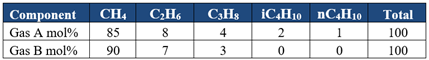Case Study: How do I size the water tank vent line to VRU compressor?

a. Process simulation

To investigate the estimation of the vent line size of the water tank, we will consider the production process flow diagram of Figure 1 (FPS). The SI version of Figure 1 (SI) is in Appendix A. the simulation results are obtained from ProMax  using the Soave-Redlich-Kwong equation of state.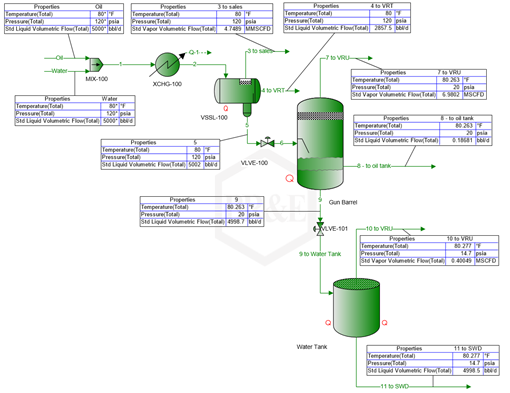Figure 1(FPS). Case study process flow diagram simulation results by ProMax 

The oil compositions and the heavy end components properties are presented in Table 2.

Table 2. Case study oil compositions and the heavy ends properties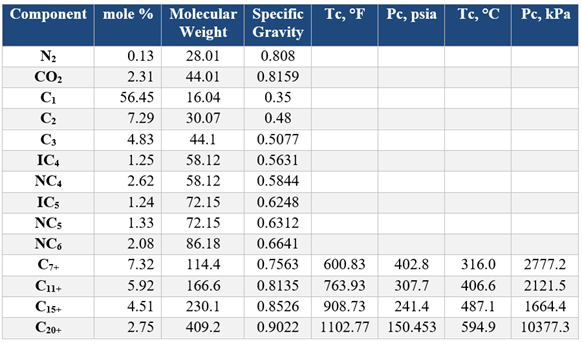As shown in Figure 1, 5000 stock tank bbl/day (33.122 STm3/h) of oil with the compositions shown in Table 2 is mixed with 5000 bbl/day (33.122 m3/h) of water at 80 °F and 120 psia (26.8 °C, 827.4 kPa) enters the three-phase separator (VSL-100). The separated water containing the dissolved and entrained hydrocarbons (stream 6) enters the gun barrel from which the separated water (Stream 9) goes to the water tank. Finally, the water (Stream 11) at a rate of 4,998.5 bbl/day (33.113 m3/h) goes to the salt-water disposal (SWD) facilities.  The tank vapor stream at the rate of 400.49 SCF/day (11.3406 Sm3/d) goes the VRU system. The solution gas, RS (Stream 9 to water tank) is 400.49 scf/4,998.5 bbl or RS = 0.0801 scf/bbl (RS =11.3406/33.113x24 = 0.01427 scm/m3).

a. Vapor recovery unit (VRU)

Figure 2 presents the schematic of water tank and its VRU system. The VRU Compressor and Scrubber have already been evaluated and are suitable for the forecasted production.  The vapor recovery line connecting the tank to the VRU compressor has not yet been evaluated. What size Vapor Recovery Line is adequate to handle the vapors generated by the inflow of water?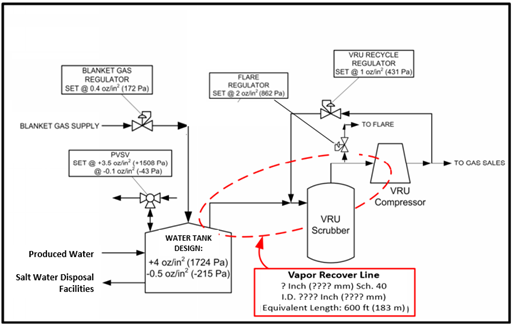Figure 2. The schematic of water tank and its VRU system

Production Conditions

Water Rate: 6,000 STBWPD (954 STm3/d)

The solution gas in feed to water tank is RS = 0.0801 scf/bbl of water (RS = 0.01427 scm/m3)

Upstream Gunbarrel Operating Pressure: 5 psig (35 kPag)

Upstream Separator Operating Temperature: 80°F (27°C)

►It is common to allow the water level in the Water Storage Tank to build up over time, thus the pump out rate from the Water Tank to the Salt Water Disposal (SWD) facilities is often intermittent.

►To determine the VRU line size, assume that the tank is in filling mode, thus the pump out rate from the tank is zero, but production from the upstream separator continues.  Total gas flow is then equal to the volume of water entering the tank (pushing the same volume of the gas out of tank), plus the gas that will evolve from the produced water (0.0801 scf/bbl or 0.01427 scm/m3).

►For the VRU line size, we also need to consider the case when water rate to SWD is the same as production from the upstream separator. Total gas flow is then equal to the volume of the gas that will evolve from the produced water (0.0801 scf/bbl or 0.01427 scm/m3)).

►During tank pump-out operations, the blanket gas system will be designed to accommodate the water removal rate from the tank, which is a different calculation than the VRU line sizing

Spitzglass formula is: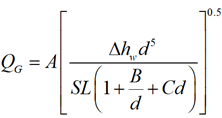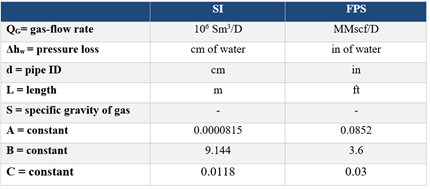►Rearranging to solve for head loss (Δhw):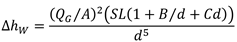FPS SOLUTION

Assume that the water flowrate is equivalent to Stock Tank flow (the temperature (80°F) is slightly higher than ‘stock tank’ (60°F). (i.e. assume Qwater = 6000 bbl/d)

QG = Qwater + Qwater*Rs, where Rs is released gas.

Therefore, gas evolving from the tank due to full water rate but zero output while the tank is filling (water tank pumps are not running):

QG = 6,000(bbl/d) x5.615(scf/bbl) +6,000(bbl/d) x 0.0801(scf/bbl) = 34,171 scfd = 0.034171 MMscfd

Let us try, ID= 2.469 inches (62.7 mm), L = 600 ft (182.9 m), and API 2000  recommends S = 1.5; therefore, this value was used even though the estimated gas relative density was S=0.84.

Substituting in: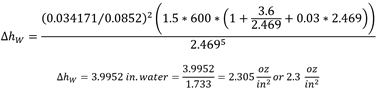Assuming the pressure at the VRU compressor suction is 1 oz/in2, the pressure at the tank would have to be 3.3 oz/in2.  This is less than the design pressure of the tank, and it is also less than the PVSV opening pressure (set at 3.5 oz/in2).

Next check if the system will handle the gas assuming that the water flow rate out of the tank is equal to the water flow rate coming into the tank.  If (QW)IN = (QW)OUT, then the gas rate is a result of gas coming out of solution so:

QG =RS * QW =0.0801 scf/bbl x 6,000 bbl/d = 481 scfd = 0.000481 MMscfd

Substituting back into Spitzglass yields: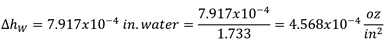This pressure is too small to be of concern. If the pressure at the VRU compressor is 1 oz/in2, then pressure at the tank would be slightly more than 1 oz/in2.  This is less than the set point of the flare valve (2 oz/in2), and less than PVSV opening pressure (set at 3.5 oz/in2). The VRU compressor is going to run at very low rates frequently and the compressor vendor would need to verify that the selected compressor can operate at all anticipated operating conditions of the system

SI SOLUTION

Assume that the water flowrate is equivalent to Stock Tank flow (the temperature (27°C) is slightly higher than ‘stock tank’ (15°C). (i.e. assume Qwater = 954 m3/d).

QG = Qwater + Qwater*Rs, where Rs is released gas.

Therefore, gas evolving from the tank due to full water rate but zero output while the tank is filling (water tank pumps are not running):

QG = 954 m3/d + 954 m3/d x 0.01427 scm/m3= 968scm/d = 0.000968x106 scm/d

Let us try, ID= 2.469 inches (62.7 mm), L = 600 ft (182.9 m), and API 2000  recommends S = 1.5; therefore, this value was used even though the estimated gas relative density was S=0.84.

Substituting in: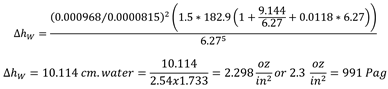Assuming the pressure at the VRU compressor suction is 431 Pa (1 oz/in2), the pressure at the tank would have to be 1.422 kPag (3.3 oz/in2).  This is less than the design pressure of the tank, and it is also less than the PVSV opening pressure (set at 1.509 kPag = 3.5 oz/in2).

Next, check if the system will handle the gas assuming that the water flow rate out of the tank is equal to the water flow rate coming into the tank.   If (QW)IN = (QW)OUT, then the gas rate is a result of gas coming out of solution so:

QG =RS * QW =954 m3/d x 0.01427 scm/m3= 13.6136 scm/d = 0.000014x106 scm/d

Substituting back into Spitzglass yields: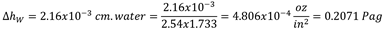This pressure is too small to be of concern. If the pressure at the VRU compressor is 431 Pa (1 oz/in2), then pressure at the tank would be slightly more than 1 oz/in2.  This is less than the set point of the flare valve (862 Pag = 2 oz/in2), and less than PVSV opening pressure (set at set at 1.508 kPag = 3.5 oz/in2). The VRU compressor is going to run at very low rates frequently and the compressor vendor would need to verify that the selected compressor can operate at all anticipated operating conditions of the system.

Table 3 shows the comparison of pressure drops estimated by three correlations and indicates that the Spitzglass value is the most conservative.

Table 3. Vent line pressure drop by three correlations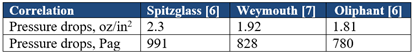Summary

This TOTM presented the computer simulation results for an oil production facility (Figure 1) with a watercut of 50 % by volume. From the simulation result, the produced water rate and the vent gas rate from the water tank were used to estimate the diameter of the vent pipe to the VRU compressor (Figure 2). The diameter was estimated by the Spitzglass correlation under pressure setting constrained shown in Figure 2. In addition, the vent line pressure drop was estimated by Weymouth and Oliphant correlations (Table 3). Table 3 indicates that the Spitzglass value is the most conservative. This is important because often, these systems operate with very tight tolerances.  Notice that when the saltwater disposal system is not removing water from the tank (our design case here), the pressure in the tank is 3.3 oz/in2 [1.422 kPag] and the opening pressure for the PVSV is 3.5 oz/in2 [1.422 kPag].  This provides very little margin for error, justifying the use of the most conservative algorithm (e.g. Spitzglass).  It also highlights the importance of designing this vapor line with ample drainage, and “No Pockets” or traps where liquids can accumulate.

To learn more about similar cases and how to minimize operational problems, we suggest attending our G4 (Gas Conditioning and Processing), G5 (Practical Computer Simulation, and Applications in Gas Processing) courses.

By: Mahmood Moshfeghian, Ph.D., & Kindra Snow-McGregor, P.E.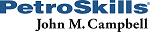References:

1. Sloan, E.D., Koh, C.A., Clathrate Hydrates of Natural Gases, 3rd ed., CRC Press, 2008.

2. Moshfeghian, M., http://www.jmcampbell.com/tip-of-the-month/2021/01/solubility-of-gases-in-water-part-1/, Light Hydrocarbon gases, Jan 2021

3. Moshfeghian, M., https://www.petroskills.com/blog/entry/00_totm/mar21-fac-Solubility-of-Gases-in-WaterP2#.YFoq4VVKipo , Mar 2021

4. ProMax 5.0, Build 5.0.20034.0, Bryan Research and Engineering, Inc., Bryan, Texas, 2020.

5. API Standard 2000, Venting Atmospheric and Low-Pressure Storage Tanks, 7th Edition, American Petroleum Institute. 2014

6. GPSA Engineering Data Book, Section 17, Volume 2, 13th Edition, Gas Processors and Suppliers Association, Tulsa, Oklahoma, 2012.

7. Campbell, J.M., “Gas Conditioning and Processing, Volume 1: The Fundamentals,” 9th Edition, 3rd Printing, Editors Hubbard, R. and Snow–McGregor, K., Campbell Petroleum Series, Norman, Oklahoma, PetroSkills 2018

Appendix A

Vent gas rate is QG = 34171 scfd (From FPS Solution section)

Oliphant Correlation 

Weymouth Correlation 

Figure 1(SI). Case study process flow diagram simulation results by ProMax 

## Sidebar

### Popular tags

#Renewables #LNG #Unconventionals